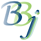#IF..THEN..ELSE Statements

## BBj-Specific Information

For this topic's original documentation, see the IF Verb.

In BBj, the IF verb supports an optional multi-line syntax for IF..THEN..ELSE statements. The statement must be terminated with fi or endif. ENDIF can be used in place of FI in any IF statement.

## Example 1

 if x=0 then  x=1  y=8endif

## Example 2

 if x=1 then  x=  y=6else  x=9  z=r fi

Also in BBj, single-line IF statements that are not terminated with an ENDIF or FI are terminated with an implicit ENDIF. Therefore, some constructs that were supported in BBx are not supported in BBj.

For example:

 IF X=1 THEN GOTO EOP ELSE FOR X=1 TO 10PRINT XNEXT XEOP:END

This construct can be re-written as:

 IF X<>1 THEN   FOR X=1 TO 10      PRINT X   NEXT XENDIFEND